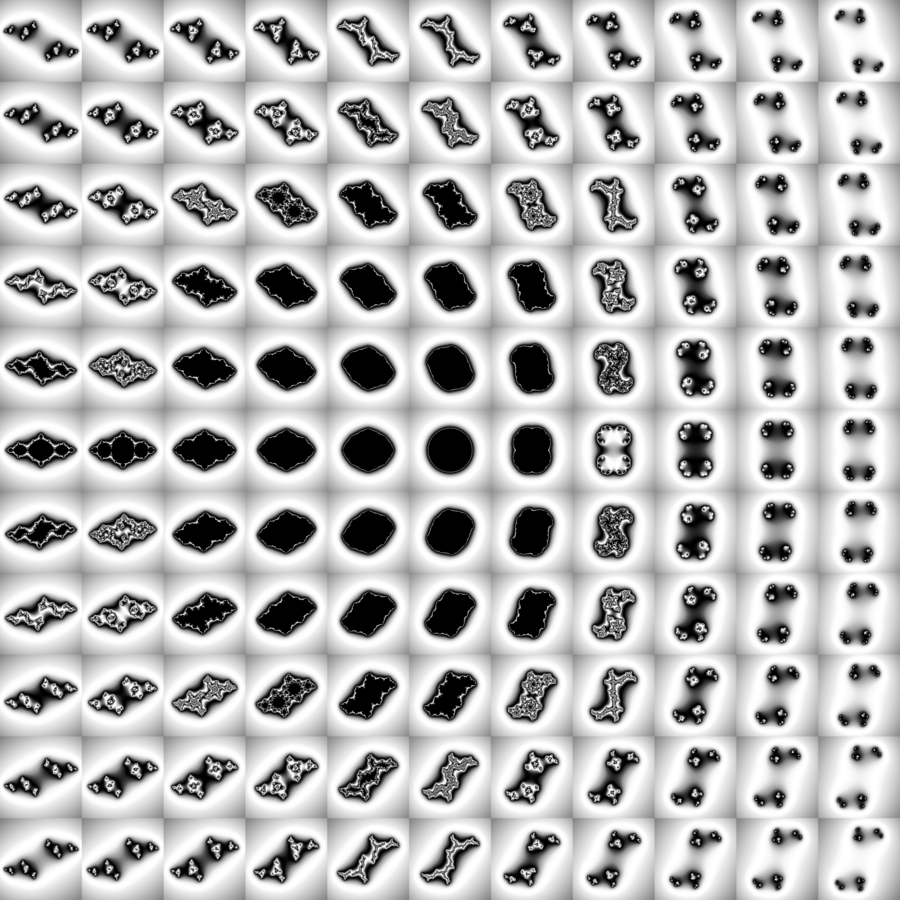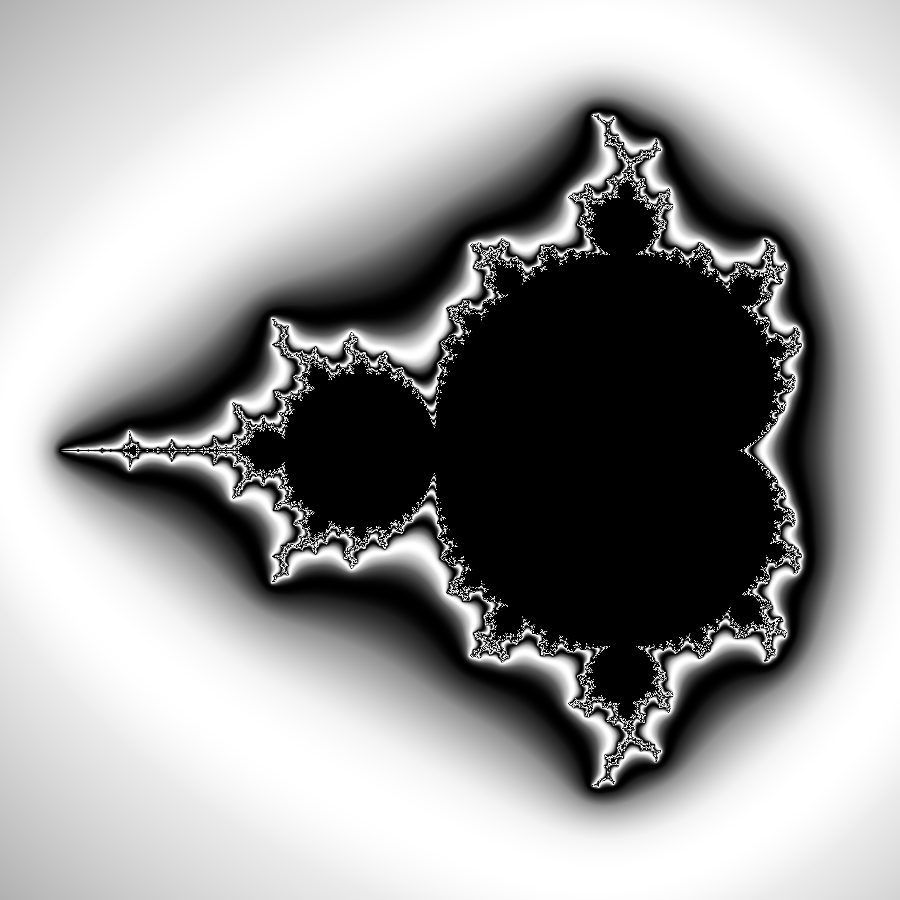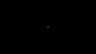# Mandelbrot and Julia

### Rendered in Python, explained in English

#### Julia

For each point in the complex plane there is a Julia set. (But there are also many others! The ones here are called 'Complex quadratic polynomial' Julia sets). The Julia set for point z0, is all points z in the complex plane, that when iterated throught f(z) = z*z + z0, stay bounded.

Below is a matrix of Julia sets in the range of -1 <= a,b <= 1, where a+bi = z0.

See the code here#### Mandelbrot

The Mandelbrot set is a varient of Julia; instead of having one z0 for the whole set, every point is calculated with its own original location as z0.

See the code hereBy rendering a lot of images, and compiling them into a gif, I've created this tiny version of a zoom-in animation into mandelbrot: (But I'm afraid I've lost the script)The small Mandelbrot thumbnail at the end of every page in this site, is rendered using this script.